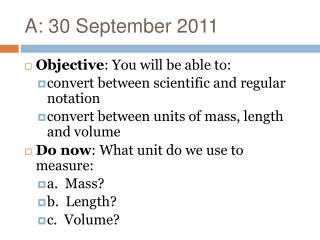Download PresentationA: 30 September 2011

# A: 30 September 2011

Download Presentation## A: 30 September 2011

- - - - - - - - - - - - - - - - - - - - - - - - - - - E N D - - - - - - - - - - - - - - - - - - - - - - - - - - -
##### Presentation Transcript

1. A: 30 September 2011 • Objective: You will be able to: • convert between scientific and regular notation • convert between units of mass, length and volume • Do now: What unit do we use to measure: • a. Mass? • b. Length? • c. Volume?

2. Agenda • Do now • Scientific notation notes and practice • Conversion between units notes and practice • Exit ticket • Homework work time?!? Homework: Week 4 Homework pages 1-4 due Tuesday Lab Notebook due Tuesday

3. Properties • Intensive: the property does NOT depend on the amount of stuff you have • ex: temperature • Extensive: the property DOES depend on the amount of stuff you have • ex: mass • Use your data to determine if density is an intensive property or an extensive property!

4. Scientific Notation • 602,000,000,000,000,000,000,000 • The number of atoms of iron in this beaker! • Scientific Notation: A way to effectively show and compare really large or really small numbers.

5. Scientific Notation • 8,000,000 = 8x106 • 0.00012 = 1.2x10-4 • Integer must be 1≤x<10 • Positive exponent: number > 1 • Negative exponent: number < 1 (but > 0!)

6. Practice • 4,500,000 • 3,950,000,000 • 230 • 230. • 0.00000045 • -0.002 • 0.00781

7. Convert to regular notation • 3.4x1012 • 5.4x10-6 • 1.2x103 • 7.80x10-2 • 1.20x10-8 • 3.98x1010 • 3x10-2

8. Copy the number and rewrite in scientific notation or regular notation • 2.3 x10-2 • 1.99 x104 • 9 x10-8 • 6.505 x10-12 • 1.00 x10-4 • 4.55 x1015 • 100,000 • -5,000,000 • 450,000,000,000 • 1,300 • 0.01 • 0.00 005 • -0.0 045 • 0.00 000 000 000 000 023

9. Remember… • A negative exponent is a tiny number but is bigger than 0 (NOT a negative number!) • A big positive exponent is a HUGE number. • A negative number can have either a positive exponent or a negative exponent.

10. Round to three sig. fig. and express in scientific notation • 745,000 • 0.00054000 • 540,321,324 • 0.143589

11. C: 3 October 2011 • Take Out Homework: Week 4 p. 1 • Objective: You will be able to: • convert between units of mass, length and volume • Do now: What unit do we use to measure: • a. Mass? • b. Length? • c. Volume?

12. Agenda • Do now (6 min.) • Homework Check (5) • SI units and prefixes notes (5) • Conversions examples (10) • Conversions practice problems (10 min.) • Go over answers (5 min.) • Exit Ticket (10 min.) Homework: Week 4 pages 2-4: due Weds.

13. Unit Conversions

14. SI Units for Measurement

15. Unit Prefixes • But what if it’s not practical to use a meter or a liter or a gram? • You want to measure something much smaller or larger?

16. SI Unit Prefixes • So what does that mean? • 1 meter = 100 centimeters • 1 kilometer = 1000 meters

17. Converting between units • Example 1: How many meters are equal to 5 kilometers? • Example 2: How many milliliters are equal to 75.0 liters? • Example 3: How many kilograms are equal to 2,500 grams?

18. C: 5 October 2011 • Take Out Homework: Week 4 p. 2-3 • Objective: You will be able to: • convert between units of mass, length and volume • differentiate between physical and chemical properties and changes. • Do now: How many centimeters are equal to 4.50 meters?

19. Agenda • Do now (6 min.) • Homework Check (5) • Conversions practice problems (10 min.) • Go over answers (5 min.) • Exit Ticket (10 min.) • Chemistry, physical and chemical changes and states of matter Homework: Week 4 page 4: Thurs. Week 5 Homework page 1: Thurs.

20. Homework Check

21. Practice Problems • 1 meter = 100 cm, 1 kilometer = 1000 meters • How many meters are equal to 500 centimeters? • How many centimeters are equal to 850 meters? • How many meters are equal to 37.5 kilometers? • How many centimeters are equal to 5.8 kilometers?

22. Practice Problems • 1 liter = 1000 milliliters • How many liters are equal to 550 milliliters? • How many milliliters are equal to 3.5 liters? • How many liters are equal to 45,000 milliliters? • How many milliliters are equal to 354 liters?

23. Exit Ticket

24. Next PowerPoint!